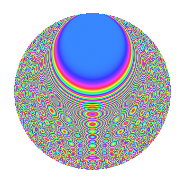# Properties

 Label 1050.2.bgLevel 1050 Weight 2 Character orbit bg Rep. character $$\chi_{1050}(121,\cdot)$$ Character field $$\Q(\zeta_{15})$$ Dimension 320 Sturm bound 480

# Learn more about

## Defining parameters

 Level: $$N$$ = $$1050 = 2 \cdot 3 \cdot 5^{2} \cdot 7$$ Weight: $$k$$ = $$2$$ Character orbit: $$[\chi]$$ = 1050.bg (of order $$15$$ and degree $$8$$) Character conductor: $$\operatorname{cond}(\chi)$$ = $$175$$ Character field: $$\Q(\zeta_{15})$$ Sturm bound: $$480$$

## Dimensions

The following table gives the dimensions of various subspaces of $$M_{2}(1050, [\chi])$$.

Total New Old
Modular forms 1984 320 1664
Cusp forms 1856 320 1536
Eisenstein series 128 0 128

## Trace form

 $$320q$$ $$\mathstrut +\mathstrut 40q^{4}$$ $$\mathstrut +\mathstrut 4q^{5}$$ $$\mathstrut +\mathstrut 8q^{6}$$ $$\mathstrut -\mathstrut 12q^{7}$$ $$\mathstrut +\mathstrut 40q^{9}$$ $$\mathstrut +\mathstrut O(q^{10})$$ $$320q$$ $$\mathstrut +\mathstrut 40q^{4}$$ $$\mathstrut +\mathstrut 4q^{5}$$ $$\mathstrut +\mathstrut 8q^{6}$$ $$\mathstrut -\mathstrut 12q^{7}$$ $$\mathstrut +\mathstrut 40q^{9}$$ $$\mathstrut +\mathstrut 2q^{10}$$ $$\mathstrut -\mathstrut 12q^{11}$$ $$\mathstrut -\mathstrut 4q^{15}$$ $$\mathstrut +\mathstrut 40q^{16}$$ $$\mathstrut +\mathstrut 24q^{17}$$ $$\mathstrut -\mathstrut 8q^{19}$$ $$\mathstrut -\mathstrut 8q^{20}$$ $$\mathstrut -\mathstrut 32q^{22}$$ $$\mathstrut +\mathstrut 12q^{23}$$ $$\mathstrut +\mathstrut 16q^{24}$$ $$\mathstrut -\mathstrut 6q^{25}$$ $$\mathstrut +\mathstrut 22q^{28}$$ $$\mathstrut -\mathstrut 48q^{29}$$ $$\mathstrut +\mathstrut 16q^{30}$$ $$\mathstrut -\mathstrut 6q^{31}$$ $$\mathstrut +\mathstrut 4q^{33}$$ $$\mathstrut +\mathstrut 32q^{34}$$ $$\mathstrut +\mathstrut 40q^{35}$$ $$\mathstrut -\mathstrut 80q^{36}$$ $$\mathstrut +\mathstrut 16q^{37}$$ $$\mathstrut +\mathstrut 16q^{38}$$ $$\mathstrut +\mathstrut 2q^{40}$$ $$\mathstrut +\mathstrut 72q^{41}$$ $$\mathstrut +\mathstrut 6q^{42}$$ $$\mathstrut +\mathstrut 144q^{43}$$ $$\mathstrut +\mathstrut 8q^{44}$$ $$\mathstrut +\mathstrut 4q^{45}$$ $$\mathstrut +\mathstrut 12q^{46}$$ $$\mathstrut -\mathstrut 40q^{47}$$ $$\mathstrut -\mathstrut 12q^{49}$$ $$\mathstrut +\mathstrut 16q^{50}$$ $$\mathstrut +\mathstrut 72q^{53}$$ $$\mathstrut -\mathstrut 4q^{54}$$ $$\mathstrut -\mathstrut 12q^{55}$$ $$\mathstrut +\mathstrut 16q^{57}$$ $$\mathstrut -\mathstrut 28q^{58}$$ $$\mathstrut -\mathstrut 24q^{59}$$ $$\mathstrut -\mathstrut 8q^{60}$$ $$\mathstrut -\mathstrut 16q^{61}$$ $$\mathstrut +\mathstrut 72q^{62}$$ $$\mathstrut +\mathstrut 20q^{63}$$ $$\mathstrut -\mathstrut 80q^{64}$$ $$\mathstrut -\mathstrut 16q^{65}$$ $$\mathstrut -\mathstrut 16q^{66}$$ $$\mathstrut -\mathstrut 16q^{67}$$ $$\mathstrut -\mathstrut 16q^{68}$$ $$\mathstrut +\mathstrut 32q^{69}$$ $$\mathstrut +\mathstrut 22q^{70}$$ $$\mathstrut +\mathstrut 64q^{71}$$ $$\mathstrut -\mathstrut 24q^{73}$$ $$\mathstrut -\mathstrut 32q^{74}$$ $$\mathstrut +\mathstrut 8q^{75}$$ $$\mathstrut -\mathstrut 64q^{76}$$ $$\mathstrut +\mathstrut 80q^{77}$$ $$\mathstrut -\mathstrut 32q^{78}$$ $$\mathstrut -\mathstrut 12q^{79}$$ $$\mathstrut +\mathstrut 4q^{80}$$ $$\mathstrut +\mathstrut 40q^{81}$$ $$\mathstrut -\mathstrut 64q^{82}$$ $$\mathstrut +\mathstrut 88q^{83}$$ $$\mathstrut -\mathstrut 96q^{85}$$ $$\mathstrut +\mathstrut 24q^{86}$$ $$\mathstrut +\mathstrut 28q^{87}$$ $$\mathstrut -\mathstrut 14q^{88}$$ $$\mathstrut -\mathstrut 4q^{90}$$ $$\mathstrut +\mathstrut 68q^{91}$$ $$\mathstrut +\mathstrut 16q^{92}$$ $$\mathstrut +\mathstrut 32q^{93}$$ $$\mathstrut -\mathstrut 16q^{94}$$ $$\mathstrut +\mathstrut 68q^{95}$$ $$\mathstrut -\mathstrut 4q^{96}$$ $$\mathstrut +\mathstrut 60q^{97}$$ $$\mathstrut +\mathstrut 32q^{98}$$ $$\mathstrut -\mathstrut 16q^{99}$$ $$\mathstrut +\mathstrut O(q^{100})$$

## Decomposition of $$S_{2}^{\mathrm{new}}(1050, [\chi])$$ into irreducible Hecke orbits

The newforms in this space have not yet been added to the LMFDB.

## Decomposition of $$S_{2}^{\mathrm{old}}(1050, [\chi])$$ into lower level spaces

$$S_{2}^{\mathrm{old}}(1050, [\chi]) \cong$$ $$S_{2}^{\mathrm{new}}(175, [\chi])$$$$^{\oplus 4}$$$$\oplus$$$$S_{2}^{\mathrm{new}}(350, [\chi])$$$$^{\oplus 2}$$$$\oplus$$$$S_{2}^{\mathrm{new}}(525, [\chi])$$$$^{\oplus 2}$$